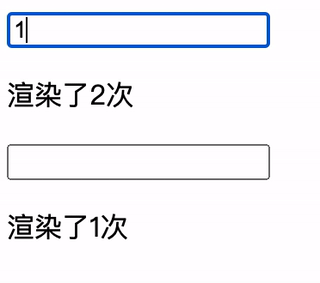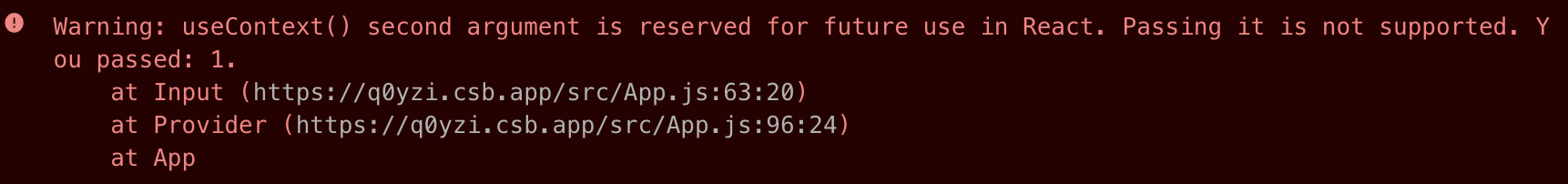# React Context精准更新

## 实现原理

``````按位或（ | ） a | b

0 | 0b01 结果为 0b01
0 & 0b01 结果为 0
``````

```const bits = {
user: 0b01,
}
```

```const context = useContext(Context, bits.user); // 这个context使用了user字段
```

`Context`的值改变的时候，会运行一个比较函数。

```const calculateChangedBits = (oldValue, newValue) => {
let result = 0;
// 标识user字段发生变化
if (oldValue.user !== newValue.user){
result |= bits.user; // 0 -> 0b01
}
result |= bits.password; // 0 -> 0b10
}
return result;
}
```

React内部会将这个`result`的值和我们在`useContext`传入的第二个参数`bits.user`进行比较。

```// observedBits 为我们使用useContext时传入的第二个参数bits.user
// changedBits 为调用比较函数后返回的值
if((observedBits & changedBits) !== 0){
// 更新该组件
}
```

## 一个例子```import { createContext, useReducer, useContext, useRef } from "react";

// 计算是那些值发生了变化
const calculateChangedBits = (oldValue, newValue) => {
let result = 0;
Object.entries(newValue.state).forEach(([key, value]) => {
if (value !== oldValue.state[key]) {
result |= bitsMap[key];
}
});
return result;
};

// 字段的标识
const bitsMap = {
user: 0b01,
};

const initialValue = { user: "", password: "" };
const reducer = (state, { name, value }) => ({ ...state, [name]: value });

// 第二个参数为比较函数
const Context = createContext(initialValue, calculateChangedBits);

const Input = ({ name }) => {
// useContext第二个参数标识该组件使用了哪一个字段
const { state, setState } = useContext(Context, bitsMap[name]);
const renderCount = useRef(0);
++renderCount.current;
return (
<div>
<input
type="text"
value={state[name]}
onChange={({ target }) => setState({ name, value: target.value })}
/>
<p>渲染了{renderCount.current}次</p>
</div>
);
};

const Provider = ({ children }) => {
const [state, setState] = useReducer(reducer, initialValue);
return (
<Context.Provider value={{ state, setState }}>{children}</Context.Provider>
);
};

export default function App() {
return (
<Provider>
<Input name="user" />
</Provider>
);
}
```

`useContext`的第二个参数除了二进制标识，也可以传`false`，表示不需要更新，这在传递`dispatch`方法的时候会很有用。

```const useDispatch = () => {
const { dispatch } = useContext(Context, false);
return dispatch;
}
```

`ContextConsumer`中使用。

```<Context.Consumer unstable_observedBits={bitsMap[name]}>
{({ state })=>{...}}
</Context.Consumer>
```

## 需要注意的地方